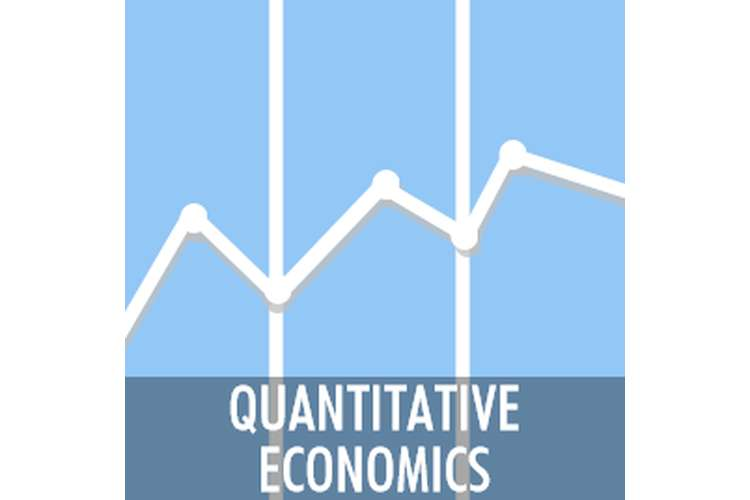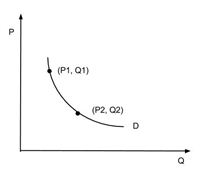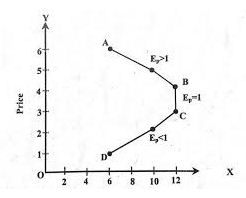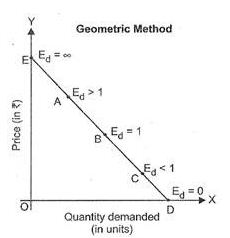# ECO82001 Economics and Quantitative Analysis## Question1

The price elasticity of demand defines or calculate the relative change in the demand for the product due change in the price of commodity other things are not changed.

### Midpoint method

The midpoint method is used as a tool for measurement of price elasticity when quantity demanded is on the bigger side due to higher change in the price of the product or commodity. The elasticity of price is then measured to take the difference between a change of quantity demanded and price of a commodity. The midpoint of the two is taken into consideration for this purpose.The price elasticity of demand can be calculated by various methods. Some of these methods are discussed below

Total outlay methodThe point methodThe percentage method

Price elasticity of Demand using midpoint method = [(Q2-Q1)/ {(Q2+Q1)/2}] / [(P2-P1)/ {(P2+P1)/2}]

#### Advantage of calculating price elasticity using Midpoint Formulae

It helps in avoiding different solution to calculate price elasticity. It helps in calculating the percentage change in quantity demanded over percentage change in the price of the product keeping in consideration the average value of both demand and price on the curve, not taking start point or end point into consideration. Further, it gives the same solution while calculating price elasticity when we move from higher price to lower price on our curve or vice-versa (Thimmapuram & Kim, 2013).

### Question 2

The price elasticity of demand is greatly affected by various factors. Some of these are discussed below

Availability of substitutes- Substitutes greatly affects the elasticity of the price for a particular product and services. If there are many substitutes for a product is there in the market then price elasticity of demand of that product will be on the higher side and vice versa (Cordera, et. al., 2015).

Many uses of a particular product- A product which has much use will have a price elasticity of demand on a higher side. Therefore the product or services provided have a single use than price is generally inelastic and vice versa (Cordera, et. al., 2015)

Nature and type of product – The nature and type of the product and services also affect price elasticity of demand. It is further classified into three types of wants – necessities, comforts and luxuries. Like goods and services of necessary items have inelastic demand while goods relating to luxuries are more elastic (Cordera, et. al., 2015).

Type of demand – the type of demand greatly affects its elasticity. For example, if the demand of the car is increased then demand of diesel or petrol will also be increased, it is complimentary in nature and like an increase in demand of an ink pen will also increase the demand of the ink (Cordera, et. al., 2015).

Level of income of the consumers of the goods – demand of the products will be inelastic for the people with high earning and good capital fund because the change in prices will not affect the demand of the product and services

Other factors include General price levels, Urgency of demand and Government policy.

### Question3

Universal music decreased average price of CD from \$21 to \$15
The quantity increase expectations of the company is 30 % of the existing sales

To calculate-price elasticity of demand for CD

Let the quantity demanded a change in prices is 100 CD’s. The price elasticity of demand can be calculated by using following formulae

ep = proportionate change in the quantity demanded/ proportionate change in price

ep= {(Q2-Q1)/Q1} / {(P2-P1)/P1}

Q1 here refers to quantity demanded before making any changes in prices that are 100

Q2 here refers to quantity demanded after making any changes in prices that are 130

P1 here refers to price of the CD’s before making any changes in prices that are \$21

P2 here refers to price of the CD’s after making any changes in prices that is \$15

ep= {(130-100)/100} / {(15-21)/21}

Price elasticity of demand is 1.05. It shows the rate of change in quantity demanded due to change in the price level of CD’s (Kyer & Maggs, 2014).

The price elasticity of demand is negative which shows the inverse relationship between price and demand of the product. The minus sign was removed from the final result because an inverse relationship is implied between the two factors. Further, it shows that for every one percent decrease in prices of the CD’s the quantity demanded will increase by 1.05 % (Kyer & Maggs, 2014).

### Question 4

The quantity demanded of these songs has been slashed by 35 %

To calculate –price elasticity of demand for the songs to be downloaded

Let the quantity demanded a change in prices is 100 downloads. The price elasticity of demand can be calculated by using following mentioned formulae

ep = proportionate change in the quantity demanded/ proportionate change in price

ep= {(Q2-Q1)/Q1} / {(P2-P1)/P1}

Q1 here refers to quantity demanded before making any changes in prices that are 100 downloads

Q2 here refers to quantity demanded after making any changes in prices that are 65 downloads

P1 here refers to price of the CD’s before making any changes in prices that are \$0.99

P2 here refers to price of the CD’s after making any changes in prices that is \$1.29

ep= {(65-100)/100} / {(1.29-0.99)/.99}

Price elasticity of demand is negative 1.155.It reflects the change in quantity demanded due to change in price level of the downloads

The negative figure of price elasticity shows the inverse relationship between price and demand of the product that is with the increase in prices of downloads the demand. The negative sign will be removed from the final result because of inverse relationship applicability between both prices and demand of the downloads. Further, it shows for every 1% increase in prices of 33 songs downloads on iTunes there will be the decrease in demand for downloads by 1.155 % (Clastres & Khalfallah, 2015).

### Question 5

A. Fall in prices of chocolate by 5%

Increase in quantity demanded of chocolate by 10%

B. The quantity demanded the ice cream has been increased by 15%

There are no changes in prices of ice cream

To find

The price elasticity of demand for chocolate sauce
Cross elasticity of demand for ice-cream with respect to price of chocolate sauce

Price elasticity of demand for chocolate sauce

ep = proportionate change in the quantity demanded/ proportionate change in price

ep= {(Q2-Q1)/Q1} / {(P2-P1)/P1}

Let the quantity demanded a change in prices is 100 that is Q1 and P1 is 100 that prices for period one (Tokarick, 2014).

ep= {(110-100)/100} / {(105-100)/100}

Price elasticity of demand is negative 2.These shows for every decrease in prices by 1 % there will be the increase in quantity demanded of the chocolate sauce by 2 %. There exist an inverse relationship between prices of the product and its quantity demanded (Tokarick, 2014).

Cross elasticity of demand for ice-cream with respect to price of chocolate sauce

Ec=percentage change in quantity demanded the product ice-cream /percentage change in quantity demanded the product chocolate sauce

Ec= 15 / 10 = 1.5.

The cross elasticity of demand for ice-cream with respect to price elasticity of chocolate sauce is 1.5. It shows when other things are equal when the prices of related goods are changed then change in quantity demanded is cross elasticity of demand

Yes, ice-cream and chocolate sauce are the complementary product to each other as a chocolate sauce is used as toppings over ice-cream. The decrease in the price of one product that is chocolate sauce will affect the demand for ice-cream also (Tokarick, 2014).

### References

Clastres, C. & Khalfallah, H. 2015, "An analytical approach to activating demand elasticity with a demand response mechanism", Energy Economics, vol. 52, pp. 195-206.

Cordera, R., Canales, C., dell’Olio, L. & Ibeas, A. 2015, "Public transport demand elasticities during the recessionary phases of economic cycles", Transport Policy, vol. 42, pp. 173-179.

Kyer, B.L. & Maggs, G.E. 2014, "Economic expansion and the balance of trade: the role of aggregate demand elasticity", American Economist, vol. 59, no. 2, pp. 176.

Thimmapuram, P.R. & Kim, J. 2013, "Consumers' Price Elasticity of Demand Modeling With Economic Effects on Electricity Markets Using an Agent-Based Model", IEEE Transactions on Smart Grid, vol. 4, no. 1, pp. 390-397.

Tokarick, S. 2014, "A method for calculating export supply and import demand elasticities", The Journal of International Trade & Economic Developmentvol. 23, no. 7, pp. 1059-1087.